# Chemistry – Nuclear Chemistry Test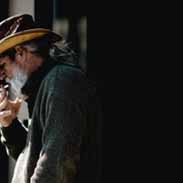Patsy Brent
question

Which radioactive nuclide is used to treat cancer?

cobalt-60
question

How many half-lives are required for three-fourths of the nuclei of one isotope in a sample to decay?

2
question

Gamma rays are

electromagnetic waves.
question

Beta particles are

electrons.
question

The half-life of an isotope is the time required for half the nuclei in a sample to

question

In a nuclear reaction, unstable nuclei change their number of protons and neutrons,

give off large amounts of energy, and increase their stability.
question

In nuclear chemistry, an atom is referred to as a

nuclide.
question

In nuclear reactors, the role of control rods is to

absorb neutrons or slow down the reaction.
question

Which statement does not describe fission?

Stable, lightweight nuclei start the process.
question

What is the function of shielding in a nuclear reactor?

question

Alpha particles are

helium nuclei.
question

What is the half-life of an isotope if 125 g of a 500 g sample of the isotope remain after 3.0 years?

1.5 years
question

A nuclide is identified by

the number of protons and neutrons in its nucleus.
question

Among atoms with low atomic numbers, what is the neutron-proton ratio of the most stable nuclei?

1:1
question

Radioactive tracers in fertilizers can be used to measure

how well the fertilizer is absorbed by plants.
question

In an artificial transmutation, what is required to bombard nuclei with positively charged alpha particles, protons, and other ions?

great quantities of energy
question

What unit measures radiation damage to human tissue?

question

The energy released in a nuclear reaction comes from

the binding energy of the nucleus.
question

question

Which of the following generally have the lowest penetrating ability?

alpha particles
question

What does the 218 in polonium-218 represent?

the mass number
question

Which of the following has the greatest penetrating ability?

gamma rays
question

OA(FL) = AL

OA = original amount, FL = fraction left, AL = amount left
question

N = log(FL) / log (.5)

N = number of half-lives, FL = fraction left
question

(1/2) ^ N = FL

N = number of half-lives, FL = fraction left
question

N = Time gone by / Time of 1 half-life

N = number of half-lives
question

Beta decay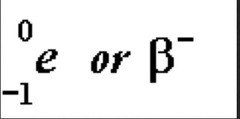question

Alpha decay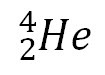question

Positron (positive beta) decay

question

Gamma decay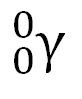question

K-capture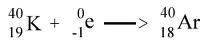EX:
question

How do nuclear power plants actually produce power/electricity?

Water is pulled in from a river/water source and turned into steam, which then drives turbine generators to produce electricity. The difference is the source of heat. At nuclear power plants, the heat to make the steam is created when uranium atoms split – called fission. Also, the nuclear aspect never comes in contact with the water/steam.
question

Mass Defect

Difference between the mass of an atom and the sum of the masses of its particles
question

Nucleon